# logarithmic spiral

## spiralThis is the spiral for which the radius grows exponentially with the angle. The logarithmic relation between radius and angle leads to the name of logarithmic spiral or logistique (in French). The distances where a radius from the origin meets the curve are in geometric progression.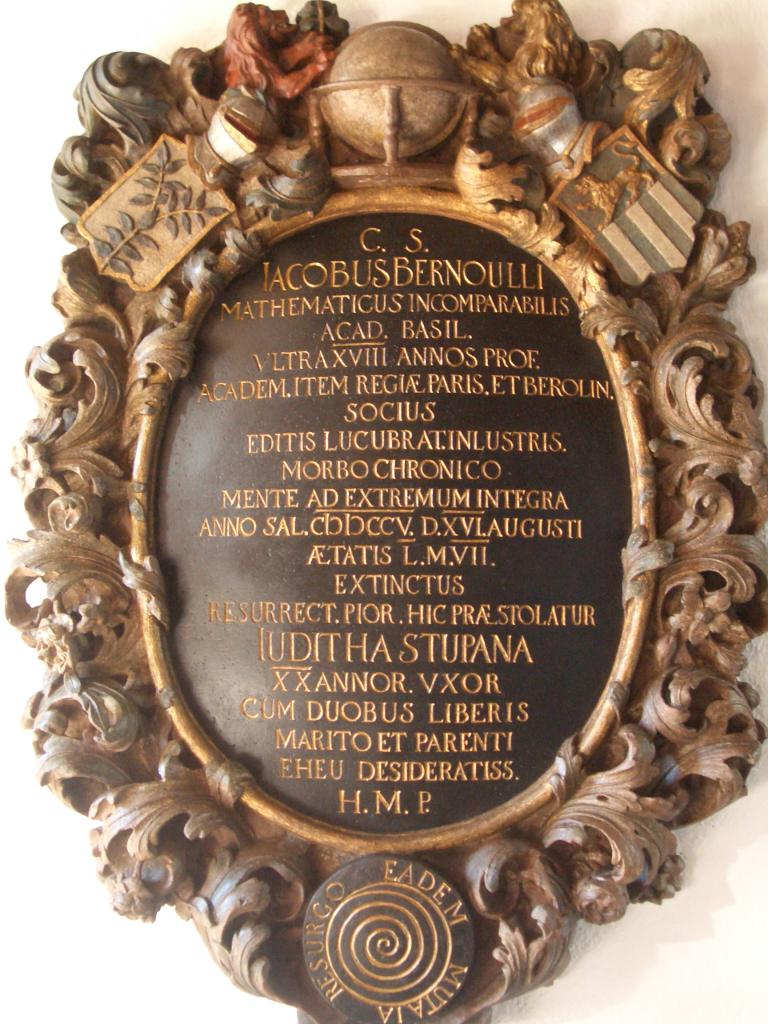The curve was the favorite of Jakob (I) Bernoulli (1654-1705). On his request his tombstone, in the Munster church in Basel, was decorated with a logarithmic spiral (bottom side). The curve, which looks by the way more like an Archimedes' spiral, has the following Latin text accompanied: eadem mutata resurgo. In a free translation: 'although changed, still remaining the same'. This refers to the various operations for which the curve remains intact (see below). Therefore the curve is also called the Bernoulli spiral.However, Rene Descartes (1638) was the first to study the curve. Torricelli worked on the curve independently, and found the curve's length. The curve is also named to Fibonacci as the Fibonacci spiral. What are the remarkable qualities of the equiangular spiral? The curve is identical to its own: Other qualities of the spiral are the following: the radius of curvature is equal to the arc length: R = s. In other words: the curvature is equal to the reciprocal arc length: dφ/ds = 1/s. when a logarithmic spiral is rolling over a line, then the path of each point of the spiral is a line the multiplication of the logarithmic spiral is equivalent with a rotation the length from the origin O to a point P(r0, φ0) of the spiral is equal to r0 sec(b), where a = cot(b) 1) Remarkable! This spiral is a real spira mirabilis, as Jakob Bernoulli called the curve in 1692.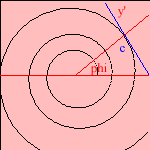The logarithmic spiral is the curve for which the angle between the tangent and the radius (the polar tangent) is a constant. Suppose that an insect flies in such a way that its orbit makes a constant angle b with the direction to a lamp. Then the poor creature travels in the form of a logarithmic spiral, eventually reaching the lamp 2). It seems that a night-moth flies under a constant angle with respect to the moon. But its orbit resembles much that of a right line, while the orbit of the moth is much smaller that the distance to the moon. This property gives the spiral the name of equiangular spiral. For angle b = π/2, the result is a circle.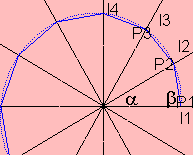The logarithmic spiral can be approximated by a series of straight lines as follows: construct a line bundle li through O with slope iα/2π. Starting with a given point P1 on l1, construct point P2 on l2 so that the angle between P1P2 and OP1 is β. Then the points Pi approximate a logarithmic spiral with a = cot β. We call the spiral curve composed of line pieces the right equiangular spiral.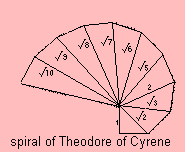For β = π/2, the line series ressembles the spiral of Theodore of Cyrene. I say "ressembles" because the Theodore of Cyrene's spiral does not define a logarithmic spiral, because not the angles, but the chords are equal (being 1). Question, what then is the equation of the spiral which the line spiral defines?When dividing a golden rectangle into squares a logarithmic spiral is formed with a = (2/π) ln φ (about 0.306), where φ is the golden ratio, with value (1+√5)/2 (about 1.62). This spiral is called the golden spiral.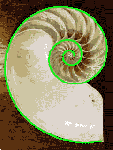We see the curve in nature, for organisms where growth is proportional to their size. An example is the Nautilus shell, where a kind of octopus hides (showed on this page). For that proportionality the curve bears the name of the growth spiral: a growth that is proportional to its size. A growth that just adds, is shown by the Archimedes' spiral. D'Arcy Thompson explains the curve in his book 'Growth and Form'. Two physical properties related to the spiral are: the force that makes a point move in a logarithmic spiral orbit is proportional to 1/r3 3). a charged particle moving in a uniform magnetic field, perpendicular to that field, forms a logarithmic spiral. Johan Gielis extended the logarithmic spiral to a super spiral.   notes 1) Remember that the arc length s can be described in polar coordinates as (ds)2 = (dr)2 +r2 (dφ)2 2) It can be proven that the desired curve is the logarithmic spiral: the curve can be found as the solution of the differential equation, which results out of the relation y' = tan(b + φ):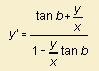Substituting y = x z and rewriting in polar coordinates gives the spiral's equation. See, for example, van der Blij 1975, p. 204. Then it follows that formula's constant a is equal to the cotangent of angle b. 3) This can be proven with help of the formula of Binet.